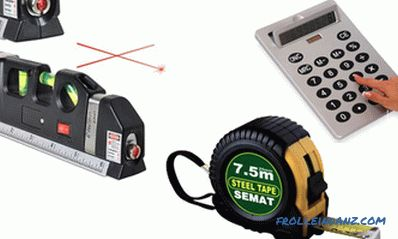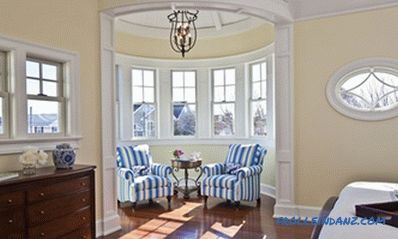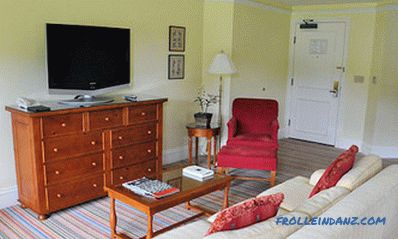# How to calculate the area of ​​the room, floor, ceiling and walls

Zatevaya repair, you must purchase a sufficient amount of wallpaper, glue, paint and other materials. To do this you need to know how to calculate the area of ​​the room. If you understand how to get and apply the necessary measurements, then in the future, for example, when buying or selling real estate, you will be able to independently calculate the floor space and check the documents.## Simple rectangular or square room

In order to learn how to calculate the floor area of ​​a room, you must determine its shape. In the room, which is a rectangle or square, you need to measure the length and width and multiply the values ​​between themselves. You will get the area of ​​the room in square meters.

The formula by which you can calculate the area:

S rooms = A x B, where A is the length, B is the width.

You can take a regular tape measure for measurement. To get the most accurate result, it is recommended to measure the length at the beginning of the wall and at the end and, if the numbers differ, take the arithmetic average.

## A room in which there are niches or protrusions

If there are niches, projections, plumbing or ventilation structures in the room, it is necessary to measure the area of ​​each section separately. If it is a niche in the wall, then the area of ​​the room must be added to the area of ​​the recess. If this is a protrusion, then, accordingly, its area will need to be subtracted from the floor space.

The same principle should be followed if you want to know how to calculate the ceiling area of ​​a room. An even, rectangular ceiling without any niches and protrusions means that it should be measured in length and width, and then calculate the area by simple multiplication.

If you plan to warm the floor with heating elements, then you will need to subtract the area occupied by heavy furniture from the total floor area.

## A room of complex configuration

Often there are rooms of unusual shape. If you can, you need to divide the room into several rectangles, calculate the area of ​​each and fold.

The area of ​​a room in the shape of a circle is calculated by the formula:

S rooms = πR², where R is the radius. It is necessary to consider how many degrees are in the sector.

To calculate the area of ​​a triangular room, use Heron’s formula:

Sc rooms = √ (P (P -A) x (P - B) x (P - C)), where R - half of the perimeter of the triangle, A, B, C - the length of its sides.

The semi-perimeter of the triangle is calculated as follows: P = (A + B + C) / 2Room with a complex configuration walls. Photo - welovead. com

## Features of calculating the area of ​​simple walls and walls containing protrusions and niches

Now let's consider such an important point as the calculation of the area of ​​the walls of a room. Knowing this value, you can buy the right amount of wallpaper for repair. Of course, you can contact a consultant in a hardware store, and he will tell you how to calculate the area of ​​the walls. There is nothing difficult in this: measure the length, width and height of the room. Next, the wall area is calculated by the formula:

Ssten = P x C, where P is the perimeter of the room, C is the height of the walls.

The perimeter of the room is calculated by the formula:

Р = (А + В) х 2, where A is the length, B is the width.

For more accurate results, the area of ​​door and window openings should be subtracted from the total area of ​​the walls.

The area of ​​the walls of a room with protrusions or indentations will differ from the area of ​​the walls of a simple room. Do not forget to add to the main value the area of ​​the wall of the protruding or in-depth area.

Be careful when making measurements and calculations. If you are using a laser tape measure, then make sure that the beam is directed strictly perpendicular to the wall, otherwise you will get a large error in the results.The walls of the room with protrusions. Photo - gohawaii. about. com